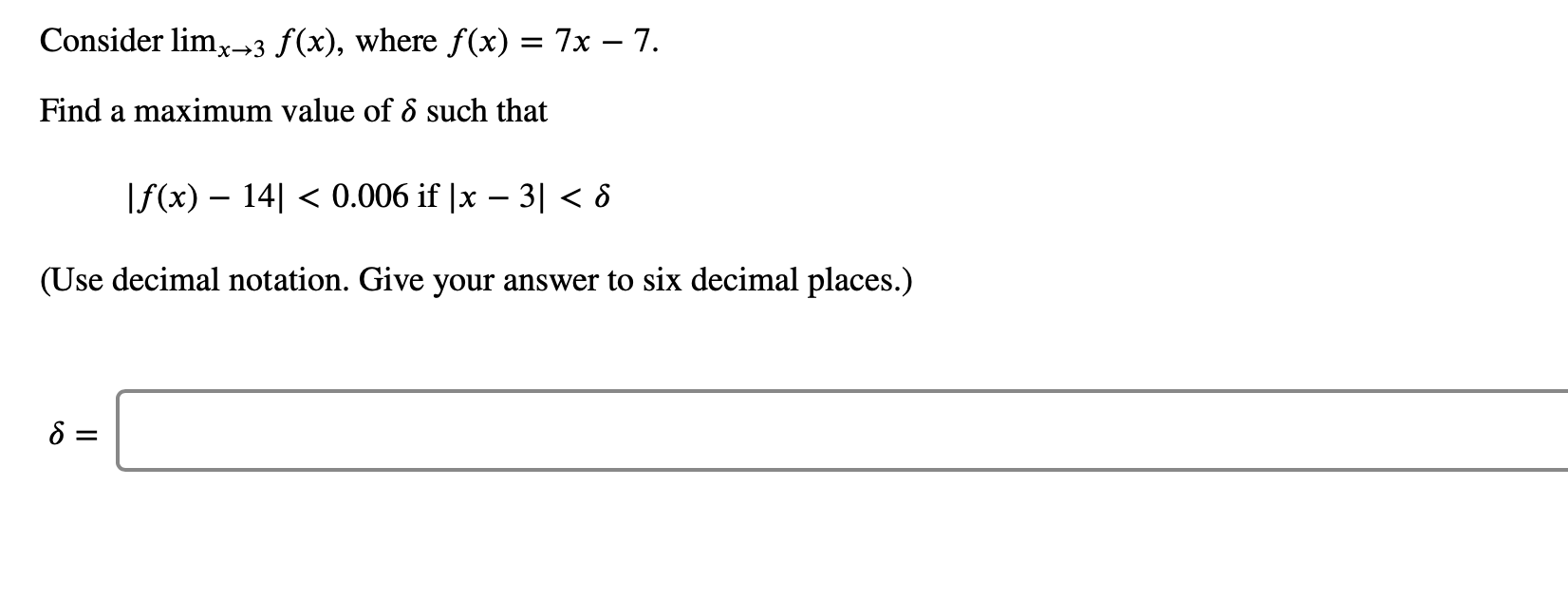# Consider limx3 f(x), where f (x) = 7x - 7.Find a maximum value of 6 such thatf(x) 140.006 if |x - 3 < 8(Use decimal notation. Give your answer to six decimal places.)

Question
1 viewshelp_outlineImage TranscriptioncloseConsider limx3 f(x), where f (x) = 7x - 7. Find a maximum value of 6 such that f(x) 140.006 if |x - 3 < 8 (Use decimal notation. Give your answer to six decimal places.) fullscreen
check_circle

Step 1

We have to plug f(x)=7x-7 i...

### Want to see the full answer?

See Solution

#### Want to see this answer and more?

Solutions are written by subject experts who are available 24/7. Questions are typically answered within 1 hour.*

See Solution
*Response times may vary by subject and question.
Tagged in

### Other